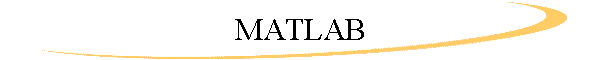on Michael Vorburger's Personal Homepage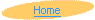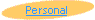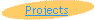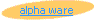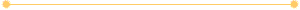These are the MATLAB scripts programmed to implement the project Face Recognition and Data Analysis as described in-depth in the scientific report.

### mtv.m

`%matrix to vector convertion`
`function [vector]= mtv(matrix)`
```[h, w]= size(matrix);
for ii= 1:h
vector((ii-1)*w+1:ii*w)= matrix(ii, :);
end```### itw.m

```% itw - image to weights
% calcs eight weight factors of a face based on nefaces```
```function [wv]=itw(fname_tiff)
global nefaces
global v```
```  [I, anymap]=tiffread(fname_tiff);
Iv=mtv(I);```
`  Iv=Iv-v;`
```  for k=1:8
wv(k)=dot(nefaces(k,:),Iv)
end```
`  wv=wv';`
```%   wv=nefaces'*Iv;
end```### line.m

```function [val]=linearize(vector,efaces)
for i=1:8;
val(i)=efaces(:,i)*vector';
end;```### line2.m

```%linearize part ii the sequel
coord=linearize(A(:,1),efaces);```
```for j=2:9;
coord=[coord; linearize(A(:,j),efaces)];
end;
``````cen=[((coord(:,1)+coord(:,2)+coord(:,3))/3)'];
for i=1:2;
cen=[cen; ((coord(:,(3*i)+1)+coord(:,(3*i)+2)+coord(:,(3*i)+3))/3)'];
end;
cen=cen';
for i=1:3;
end;
```### norma.m

```% Normalize vectors in 9xHUGE matrix

function [om]=normalize(im)
% in- & out-matrix
for i=1:9
om(i, :)=im(i, :)/norm(im(i, :) );
end```### phase1.m

```% calculate average image of all tiffs in current directory
% place average in v vector
% place vectors of remaining tiffs in A matrix
%

! /bin/ls -1 *.tiff  >directory.txt

fid= fopen('directory.txt');
counter=1;
s=fgetl(fid)
v= mtv (t);

%initializing A matrix to store picture vectors

A=v;

while (~feof(fid))

s=fgetl(fid)
if (s==-1) break;
else
% storing new tiff vector in A
A=[A;mtv(t)];

counter=counter+1

end
end
fclose(fid);
%averaging thing
v=((sum(A))/counter);

%Make A a list of the distances of the vectors of the average
for i=1:counter
A(i,:)=A(i,:)-v;
end

%Now to create C=A'A
%***C=A'*A;
%Now get the eigenvectors and we're done

%***[E,D]=eig (C);

%***[D,i]=sort(diag(D)');
%***D=fliplr(D);
%***V=fliplr(V(:,i));

% ATTENTION !!! TO BE DELETED LATER
% added to run the program in the background
% the results will be saved in the file 'matlab.mat'
% and then 'quit' will terminate matlab's process.

save
%quit

%Phase 1 Complete```### phase1a.m

```% calculate average image of all tiffs in current directory
% place average in v vector
% place vectors of remaining tiffs in A matrix
%

! /bin/ls -1 *.tiff  >directory.txt

fid= fopen('directory.txt');
counter=1;
s=fgetl(fid)
v= mtv (t);

%initializing A matrix to store picture vectors

A=v;

while (~feof(fid))

s=fgetl(fid)
if (s==-1) break;
else
% storing new tiff vector in A
A=[A;mtv(t)];

counter=counter+1

end
end
fclose(fid);
%averaging thing
v=((sum(A))/counter);
avgimg=vtm(v);
%Make A a list of the distances of the vectors of the average
for i=1:counter
A(i,:)=A(i,:)-v;
end

%Now to create C=A'A
C=A*A';
%Now get the eigenvectors and we're done

[E,D]=eig (C);

[D,i]=sort(diag(D)');
D=fliplr(D);
E=fliplr(E(:,i));
eigenfaces=E*A;

% ATTENTION !!! TO BE DELETED LATER
% added to run the program in the background
% the results will be saved in the file 'matlab.mat'
% and then 'quit' will terminate matlab's process.

save
%quit

%Phase 1 Complete```### vtm.m

```% vector to matrix conversion
% The vector is supposed to be a linearized 512*352 (rows*cols) matrix
% SIZE IS HARDCODED!```
```function [matrix]= vtm(vector)
% wv= size(vector,2);
w=352;
h=512;
for ii= 1:h
matrix(ii, :)=vector((ii-1)*w+1:ii*w);
end```### wti.m

```% wti - weights to image
% calcs an image (matrix) out of weight coords; using nefaces & v.
% weight has size 8:1```
`% global nefaces v`
```function [oi]=wti(wm)	% outimage, weightmatrix
global nefaces
global v
global map```
```    ov=wm'*nefaces;
ov=ov+v;
oi=vtm(ov);
image(oi)
colormap(map)
end```### zoom.m

`cenrad;`
```d=0
num= zeros(1,3);
for i=1:3
num(i)=norm(new_weights-cen(:,i))
end
[num, idx]= sort(num);```
```  i= idx(1);
if (i==1)
d='amir'
end;
if (i==2)
d='menashe'
end;
if (i==3)
d='yaakov'
end;```
```if d==0 d='no one'
end;```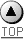Page last modified on 03-Mai-98 © Copyright 1998-99 homepage@vorburger.ch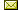Site hosted by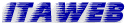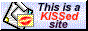KISSfp FrontPage URL: http://www.vorburger.ch/projects/faces/matlab.html